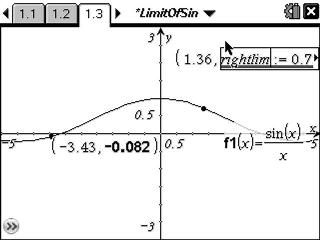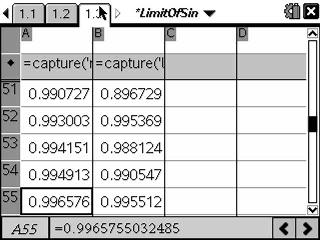# Activities

••• ##### Subject Area

• Math: Calculus: Limits of Functions

• ##### Author9-12

15 Minutes

• ##### Device
• TI-Nspire™ Navigator™
• TI-Nspire™ CAS
• ##### Software

TI-Nspire™ CAS

2.0

## Limit of Sin(x)/x#### Activity Overview

In this activity, students will graph f(x)=sin(x)/x in order to visually determine the limit as x approaches zero. They will confirm the answer numerically by tracing left and right limit points to capture values in a spreadsheet.

#### Key Steps

•In this activity, students will graph the function f (x) = sin(x)/xin order to visually determine the limit as x approaches zero.

•Students set up automated data capture and numerically investigate the values. In order to emphasize that for a limit to exist it must be approaching the same value from both sides, students will examine both sides of zero.